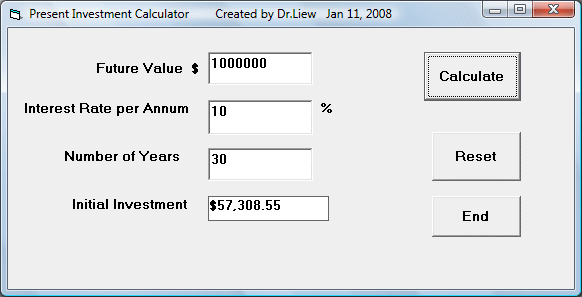# Investment Calculator

The concept of future value is related to time value of money. For example, if you deposit your money in a bank for a certain period of time, you will earn a certain amount of money based on the compound interest. This interest is computed periodically, and it is added to the principal. Interest for the following period is computed based on the initial principal plus the interest (the amount which becomes your new principal). Subsequent interests are computed in the same way.

In our Future Value Calculator, we are given a known present value and we want to calculate how much will be the future value after a numbers of years compounded upon a certain interest rate. In that example, we use the formula

FV = PV * (1 + i / 100)n

To calculate the future value. However, in this example, we already has a target future value in mind and we wish to know how much money we need to invest to achieve the target based on a certain interest. Though we still can employ the same basic formula, this time we preferred to use the VB built-in present value function, or PV.

The syntax of the PV function is

PV(Rate,Nper, Pmt,FV,Due)

Rate=Interest rate

Nper=The length of period (Usually  number of years)

Pmt=Periodic payment

FV=Future Value

*Due= 1 if payment due at beginning of a period and Due=0 if payment due at the end of a period.

### The Interface### The Code

In our example, we are considering one single initial investment in order to earn a certain amount of money in the future, so Pmt is set to 0, and payment due is at the beginning of the period, so it is set at 0, the rest of the values are obtained from the users.

Private Sub cmdCal_Click()

Dim F_Money, Int_Rate, Investment As Double
Dim numYear As Single
F_Money = Val(Txt_FV.Text)
Int_Rate = (Val(Txt_Rate.Text) / 100)
numYear = Val(Txt_Year.Text)
Investment = PV(Int_Rate, numYear, 0, F_Money, 1)
Lbl_PV.Caption = Format(-Investment, "\$##,###,##0.00")

End Sub